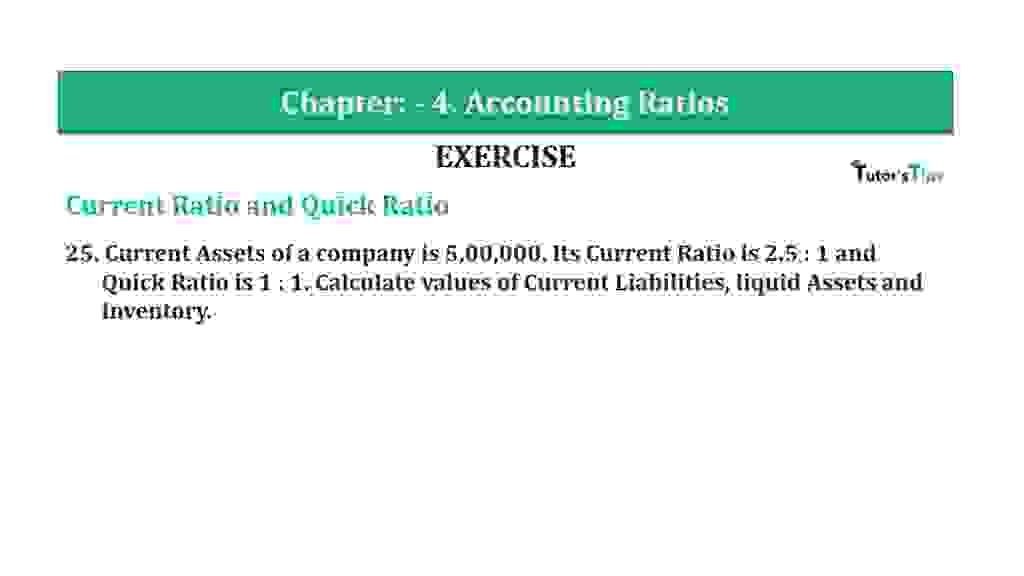# Question 25 Chapter 4 of +2-B – T.S. Grewal 12 ClassQuestion 25 Chapter 4 of +2-B

Current Ratio and Quick Ratio

25. Current Assets of a company is 5,00,000. Its Current Ratio is 2.5: 1 and
Quick Ratio is 1: 1. Calculate values of Current Liabilities, liquid Assets and Inventory.

### The solution of Question 25 Chapter 4 of +2-B: –

 Current Ratio = Current Assets = 2.5 Current Liabilities 1

 Liquid Ratio = Liquid Assets = 1 Current Liabilities 1

Current Assets  = Rs. 50,000

 Liquid Ratio = Current Assets = 5,00,000 2.5 2.5
 Liquid Assets = Current Liabilities x 1 Current Assets = Rs. 2,00,000 Inventory = Current Assets – Liquid Assets = Rs. 5,00,000 – Rs. 2,00,000 = Rs. 3,00,000

Balance Sheet: Meaning, Format & Examples

Thanks, Please Like and share with your friends

Comment if you have any question.

Also, Check out the solved question of previous Chapters: –

### T.S. Grewal’s Double Entry Book Keeping (Vol. II: Accounting for Companies)T.S. Grewal’s Analysis of Financial Statements